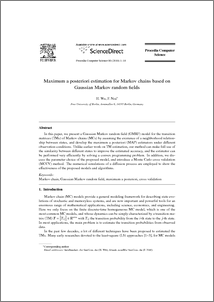Repository: Freie Universität Berlin, Math Department

# Maximum a posteriori estimation for Markov chains based on Gaussian Markov random fields

Wu, H. and Noé, F. (2010) Maximum a posteriori estimation for Markov chains based on Gaussian Markov random fields. Procedia Computer Science, 1 (1). pp. 1665-1673.Preview
PDF - Accepted Version
759kB

Official URL: http://dx.doi.org/10.1016/j.procs.2010.04.186

## Abstract

In this paper, we present a Gaussian Markov random field (GMRF) model for the transition matrices (TMs) of Markov chains (MCs) by assuming the existence of a neighborhood relationship between states, and develop the maximum a posteriori (MAP) estimators under different observation conditions. Unlike earlier work on TM estimation, our method can make full use of the similarity between different states to improve the estimated accuracy, and the estimator can be performed very efficiently by solving a convex programming problem. In addition, we discuss the parameter choice of the proposed model, and introduce a Monte Carlo cross validation (MCCV) method. The numerical simulations of a diffusion process are employed to show the effectiveness of the proposed models and algorithms.

Item Type: Article Mathematical and Computer Sciences Department of Mathematics and Computer Science > Institute of Mathematics > Comp. Molecular BiologyDepartment of Mathematics and Computer Science > Institute of Mathematics > BioComputing Group 829 BioComp Admin 07 Mar 2010 21:13 03 Mar 2017 14:40

Repository Staff Only: item control page